• 二分类预测模型
千次阅读
2019-01-18 13:59:35

本项目是数据的二分类分析，针对俄亥俄州诊所患者出现与否数据集No-show-Issue-Comma-300k进行样本的分类预测。首先进行特征探究，观察里面的连续变量，布尔值变量以及字符串变量，并绘制不同变量的分布图。然后对变量中的离群值以及明显错误数据进行清洗，探究各变量与状态变量之间的关系。最后分别用决策树，SGD，随机森林以及梯度Boosting对数据集做二分类预测，得到梯度Boosting在ROC_AUC指标下效果最好。

src="https://nbviewer.jupyter.org/github/wzy6642/Machine-Learning-Case/blob/master/noshowappointments/code/NoShowAppointments.ipynb" width="100%" height="1000">二分类预测 机器学习 数据挖掘 python 随机森林
更多相关内容
• 我们将用逻辑回归和Fisher线性判别分析两种分类算法实现二分类模型。 水果的例子： 对于因变量为分类变量的情况，我们可以使用逻辑回归进行处理。 把y看成事件发生的概率，y>0.5表示发生；y<0.5表示不...

我们将用逻辑回归Fisher线性判别分析两种分类算法实现二分类模型。

水果的例子：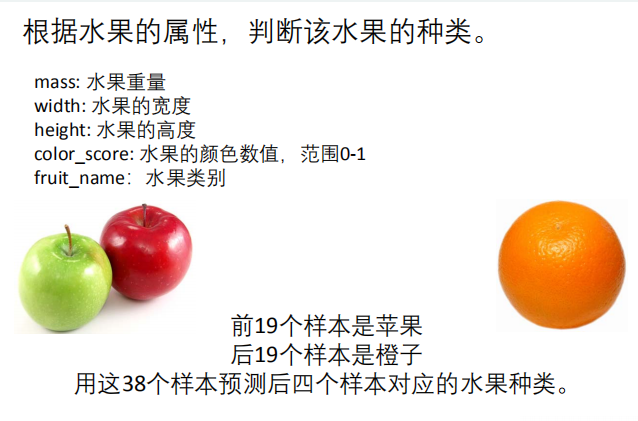对于因变量为分类变量的情况，我们可以使用逻辑回归进行处理。
y看成事件发生的概率，y>0.5表示发生；y<0.5表示不发生

逻辑回归步骤(spss)：
第一步：数据预处理：生成虚拟变量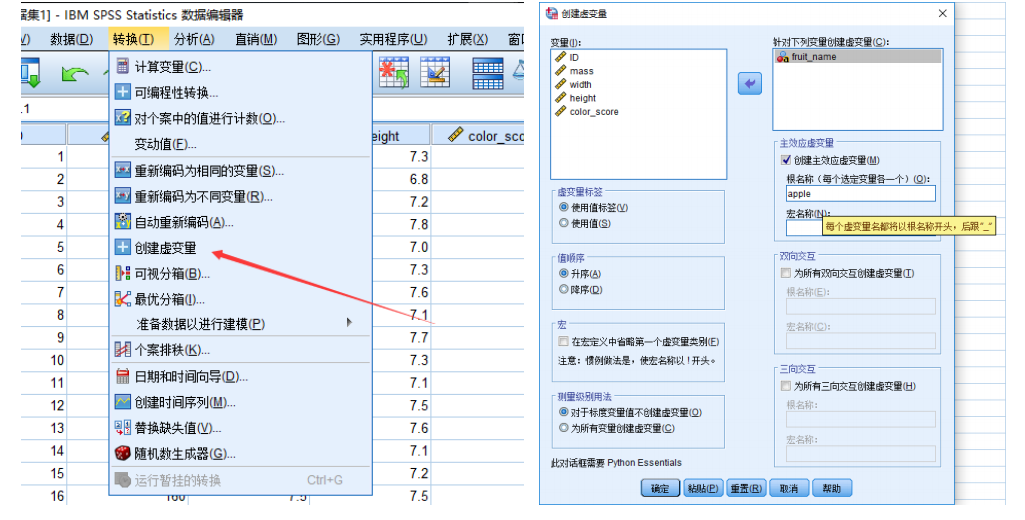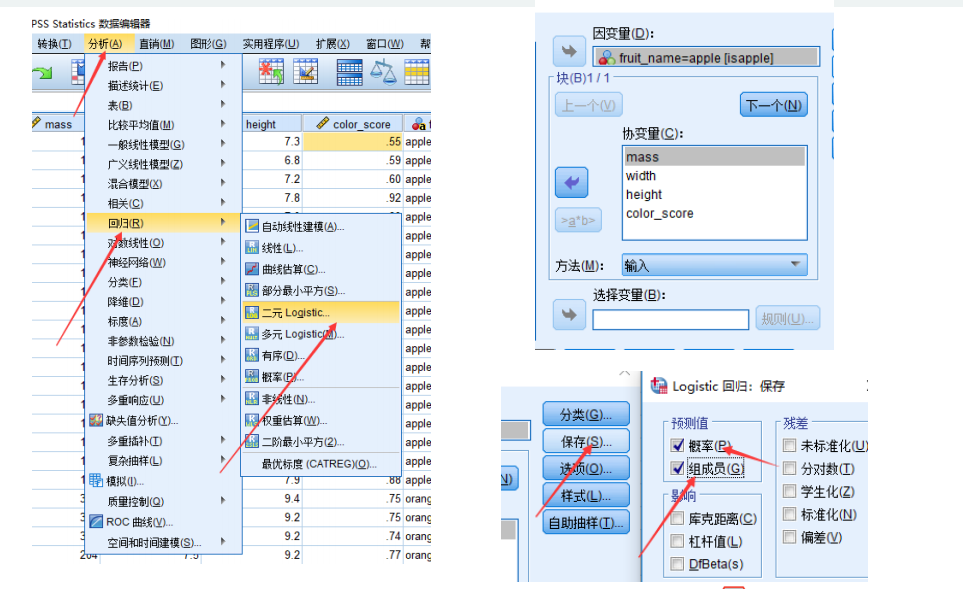预测成功率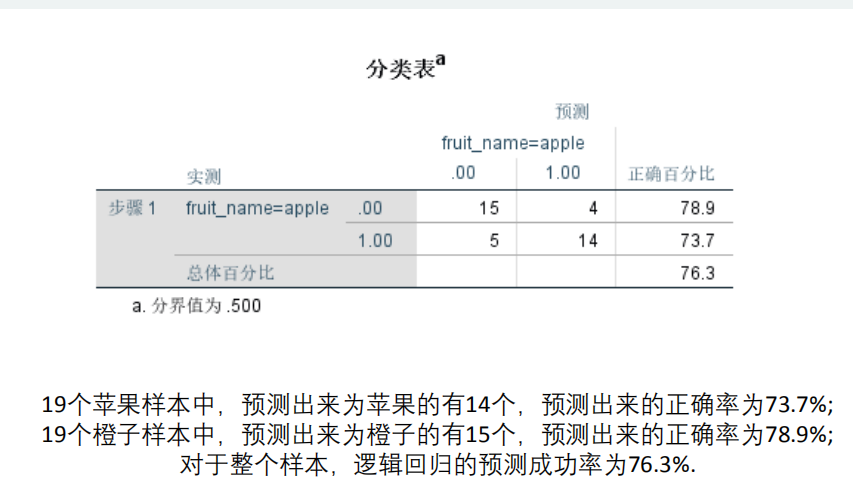逻辑回归系数表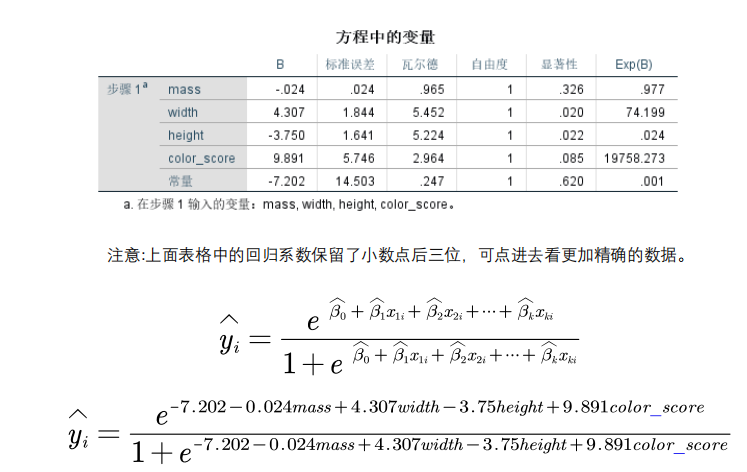表格中新添两列解读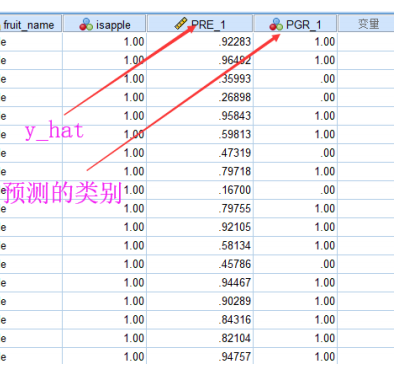y_hat:预测量

逐步回归的设置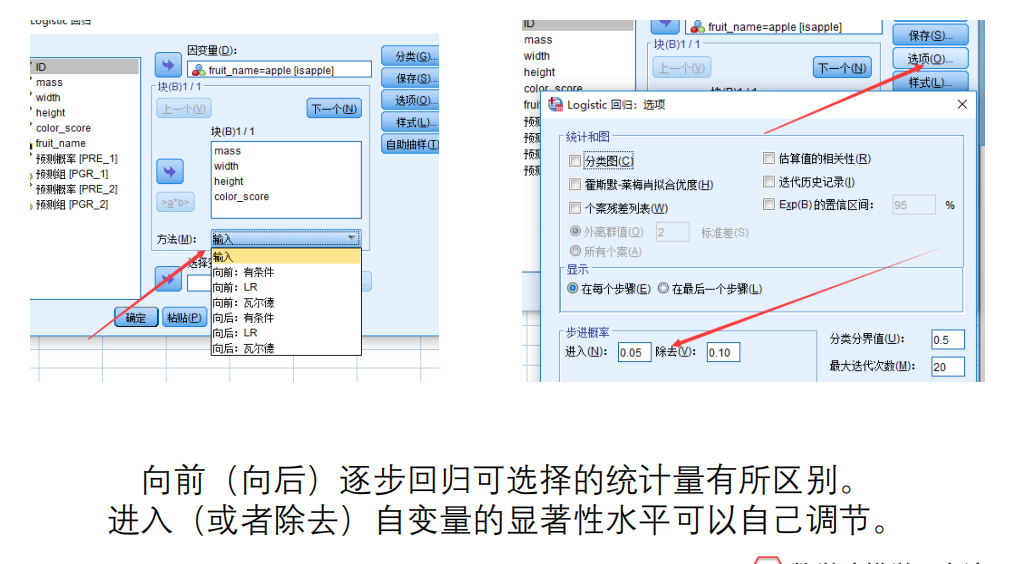假如自变量有虚拟变量怎么办？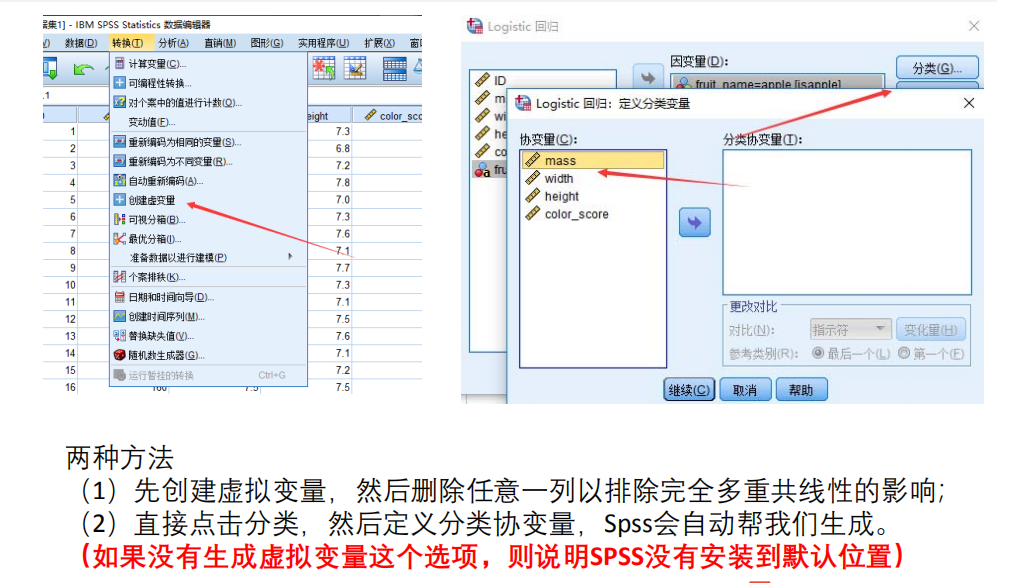预测结果较差怎么办？
可在logistic回归模型中加入平方项、交互项等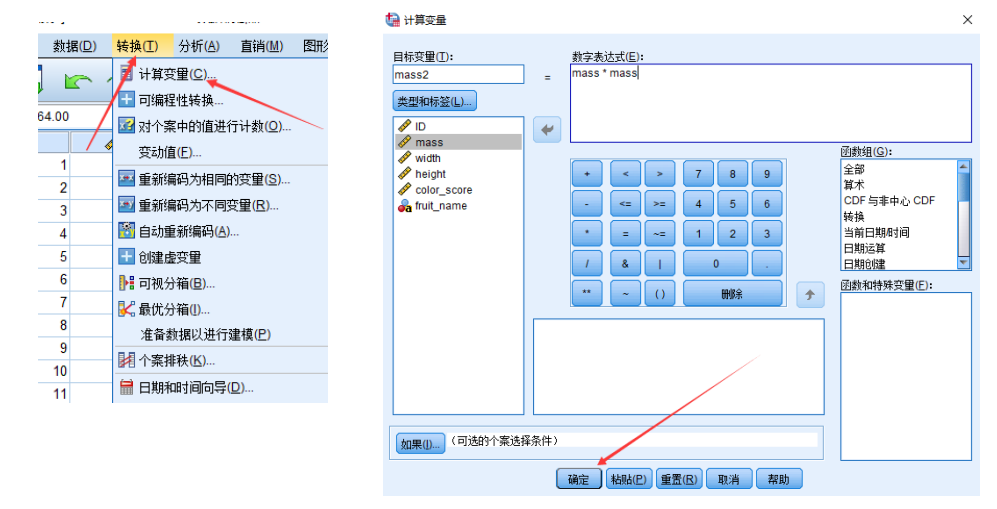加入了平方项的结果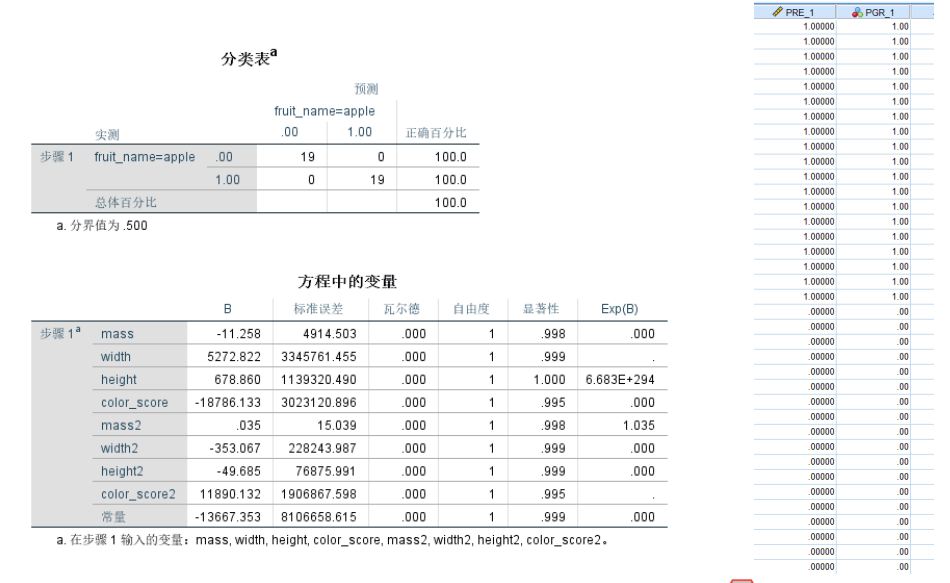加入平方项后，虽然预测能力提高了，可能会出现过拟合现象。

对于样本数据的预测非常好，但是对于样本外的数据的预测结果可能会很差。

如何确定合适的模型呢？

把数据分为训练组测试组

用训练组的数据来估计出模型，再用测试组的数据来进行测试。（训练组和测试组的比例一般设置为80% 20%)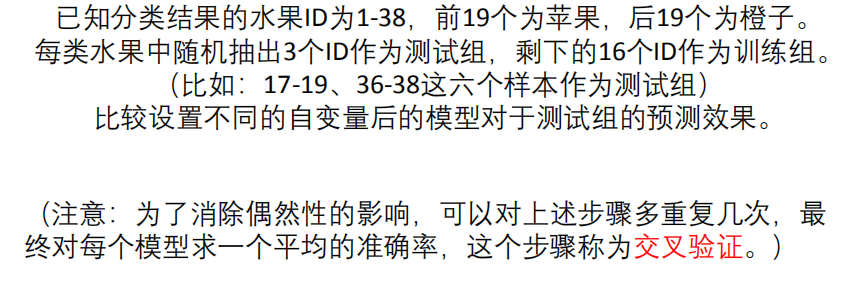Fisher线性判别分析步骤（spss）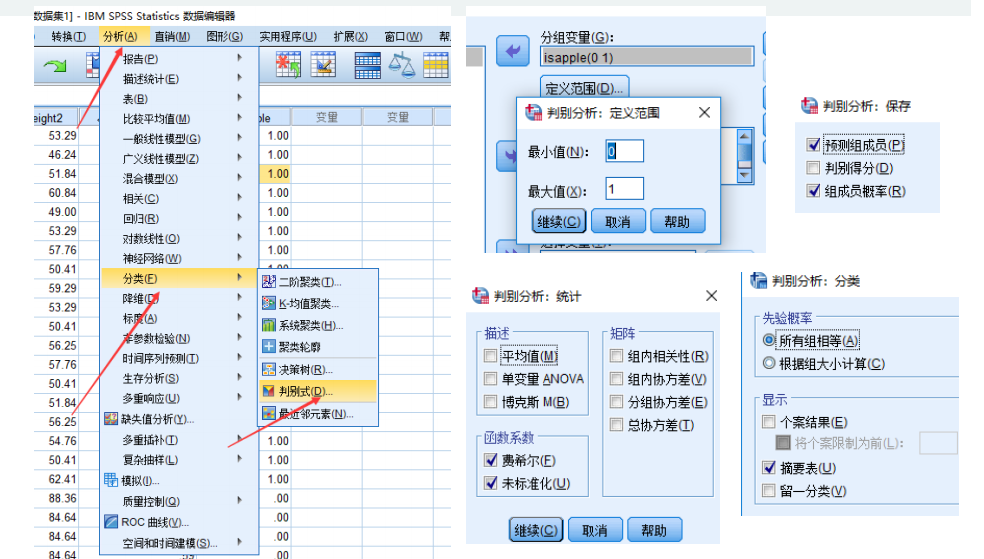结果分析：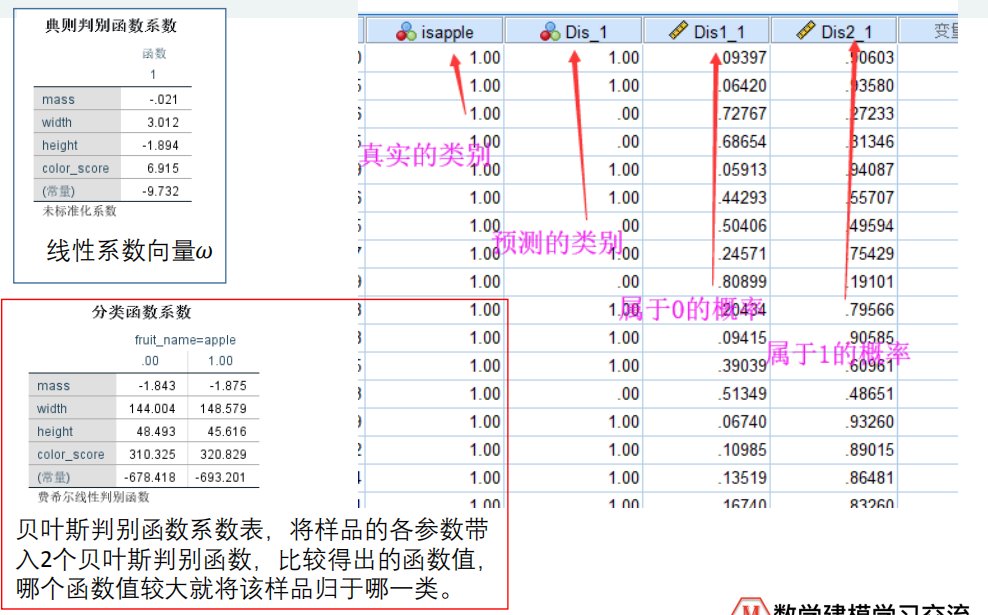多分类分析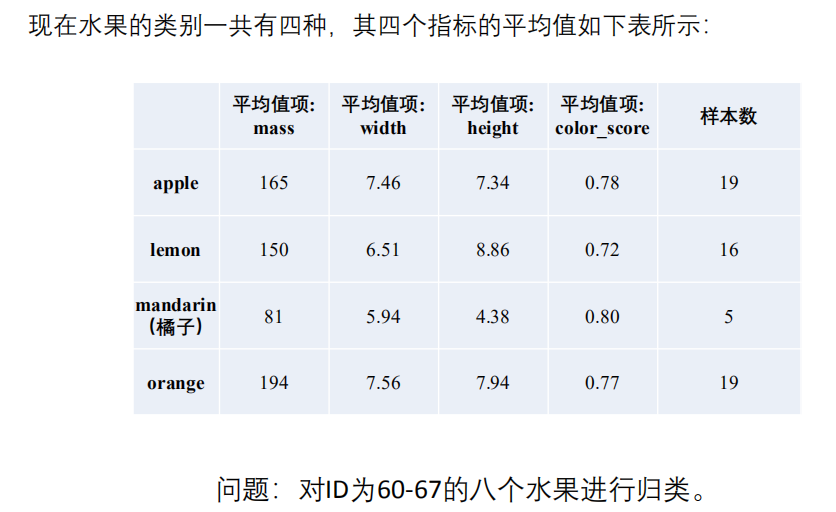Fisher判别式用于多分类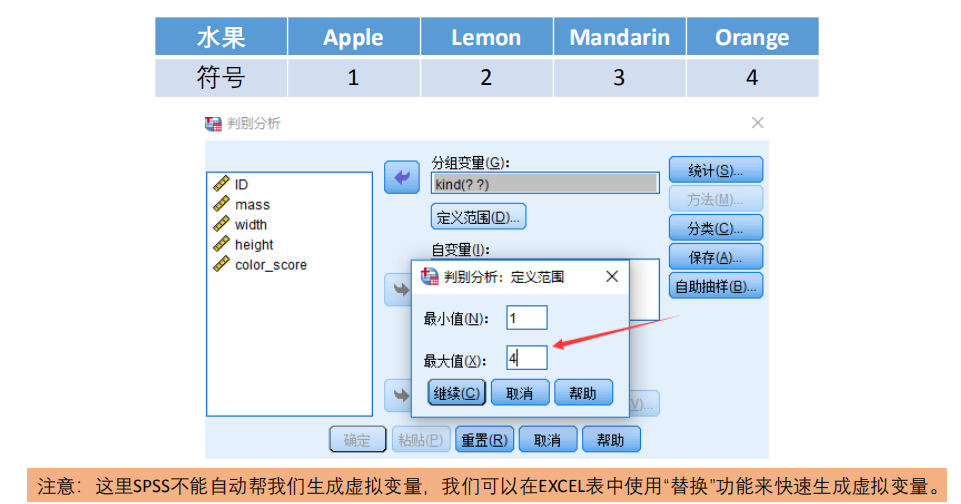结果：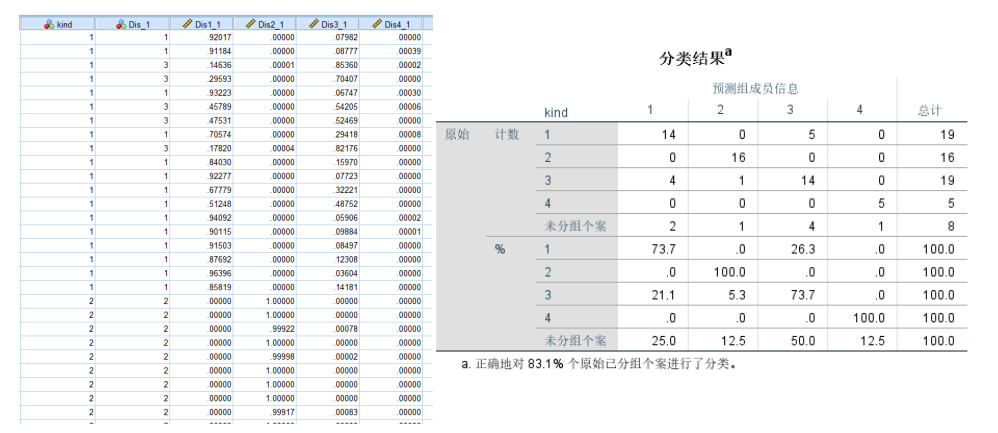展开全文• 二分类问题评价指标评价指标准确率精确率召回率F1值ROCAUC 评价指标 二分类问题评价指标的相关整理，持续更新。 评价指标是针对同样的数据，输入不同的算法，或者输入相同的算法但参数不同而给出这个算法或者参数...

## 评价指标

二分类问题评价指标的相关整理，持续更新。

评价指标是针对同样的数据，输入不同的算法，或者输入相同的算法但参数不同而给出这个算法或者参数好坏的定量指标。

二类分类问题常用的评价指标是精准度（precision）、召回率（recall）、F1值
通常以关注的类为正类，其他类为负类，混淆矩阵表示如下：TP—将正类预测为正类数
FN—将正类预测为负类数
FP—将负类预测为正类数
TN—将负类预测为负类数
得出：正样本总数 T = TP + FN, 负样本总数 F = FP + TN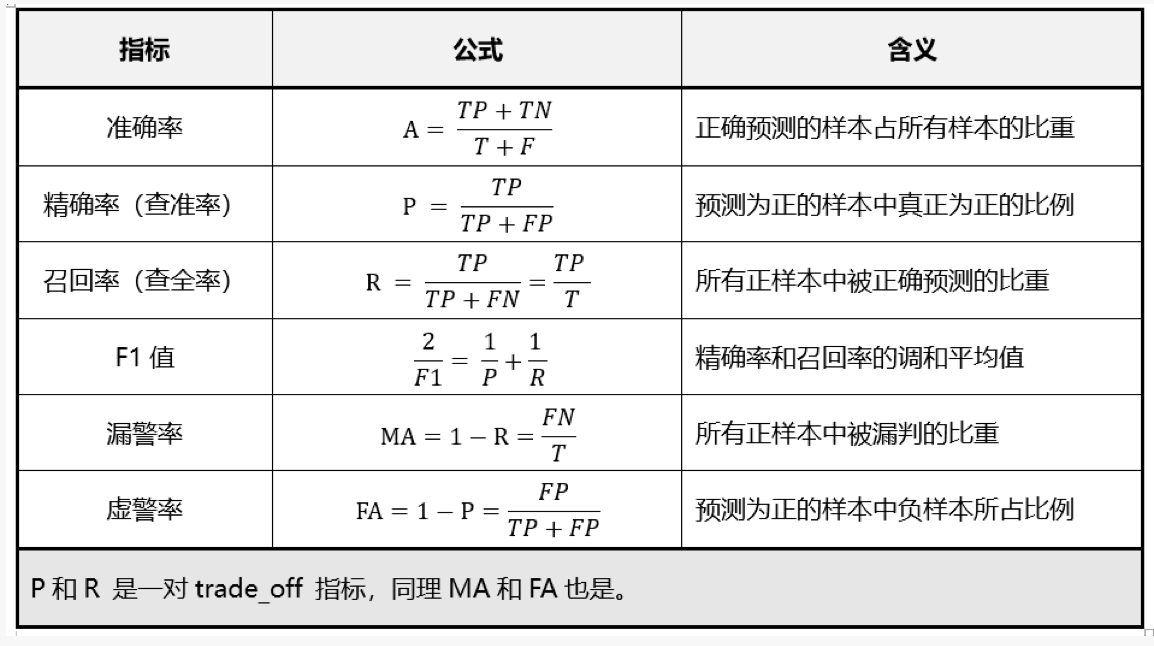## 准确率

准确率=算法分类正确的数据个数/输入算法的数据的个数
A=(TP+TN)/(T+F)
使用准确率评价算法有一个问题，就是在数据的类别不均衡，特别是有极偏的数据存在的情况下，准确率这个评价指标是不能客观评价算法的优劣的

## 精确率

精确率，预测为正的样本占所有正样本的比重
P=TP/(TP+FP)

## 召回率

正确预测的数据在总样本中的比重
R=TP/(TP+FN)=TP/T

在不同的应用场景下，我们的关注点不同，例如，在预测股票的时候，我们更关心精确率，即我们预测升的那些股票里，真的升了有多少，因为那些我们预测升的股票都是我们投钱的。而在预测病患的场景下，我们更关注召回率，即真的患病的那些人里我们预测错了情况应该越少越好

精准率和召回率是此消彼长的，即精准率高了，召回率就下降，在一些场景下要兼顾精准率和召回率，就有 F1 score

## F1值

F1 值，是精确率和召回率的兼顾指标，是精确率和召回率的调和平均数。
调和平均数的性质，只有当精确率和召回率二者都非常高的时候，它们的调和平均才会高。如果其中之一很低，调和平均就会被拉得接近于那个很低的数## ROC

ROC (Receiver operating characteristic)接收者操作特征曲线，是反映敏感性和特异性连续变量的综合指标，roc曲线上每个点反映着对同一信号刺激的感受性。
横坐标：1-Specificity，伪正类率(False positive rate， FPR)，预测为正但实际为负的样本占所有负例样本 的比例；
纵坐标：Sensitivity，真正类率(True positive rate， TPR)，预测为正且实际为正的样本占所有正例样本 的比例。

ROC曲线有个很好的特性：当测试集中的正负样本的分布变化的时候，ROC曲线能够保持不变。在实际的数据集中经常会出现类不平衡(class imbalance)现象，即负样本比正样本多很多(或者相反)，而且测试数据中的正负样本的分布也可能随着时间变化。

## AUC

AUC（Area Under Curve），是另一种评价二分类算法的指标，被定义为 ROC 曲线下的面积，这个面积的数值不会大于 1，和 F1 score 差不多，都是综合评价精准率和召回率的指标，只不过绘制 ROC 曲线使用了另外两个此消彼长的指标。
AUC的取值范围一般在0.5和1之间，使用AUC值作为评价标准是因为很多时候ROC曲线并不能清晰的说明哪个分类器的效果更好，而作为一个数值，对应AUC更大的分类器效果更好。

从AUC判断分类器（预测模型）优劣的标准：

AUC = 1，是完美分类器，采用这个预测模型时，存在至少一个阈值能得出完美预测。绝大多数预测的场合，不存在完美分类器。
0.5 < AUC < 1，优于随机猜测。这个分类器（模型）妥善设定阈值的话，能有预测价值。
AUC = 0.5，跟随机猜测一样（例：丢铜板），模型没有预测价值。
AUC < 0.5，比随机猜测还差；但只要总是反预测而行，就优于随机猜测
横坐标：假正率（False positive rate， FPR），预测为正但实际为负的样本占所有负例样本的比例。纵坐标：真正率（True positive rate， TPR），这个其实就是召回率，预测为正且实际为正的样本占所有正例样本的比例。诸如逻辑回归这样的分类算法而言，通常预测的都是一个概率值，我们会认为设置一个阈值，超过这个阈值，就预测为其中一类，不超过这个阈值，定义为另外一类。于是，不同的阈值就对应了不同的假正率和真正率，于是通过不同的阈值就形成了假正率和真正率序列，它们就可以在直角坐标系上通过描点成为光滑曲线。这个曲线就是 ROC 曲线，ROC 曲线下的面积就是 AUC。
AUC 高的算法通常认为更好。

那么为什么不用精准率和召回率画曲线求面积呢，其实是完全可以的。

精确率-召回率曲线也叫 pr 曲线，如下图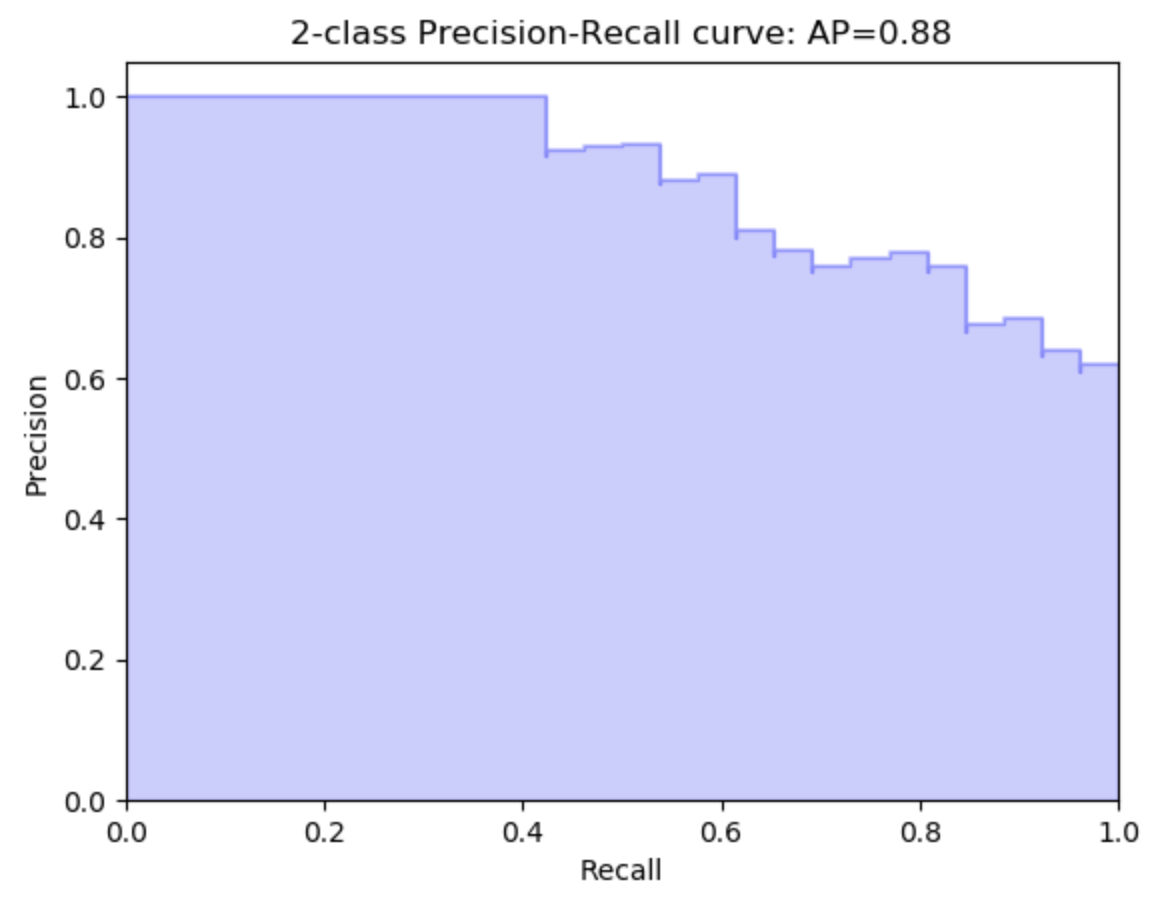而 roc 曲线，如下图：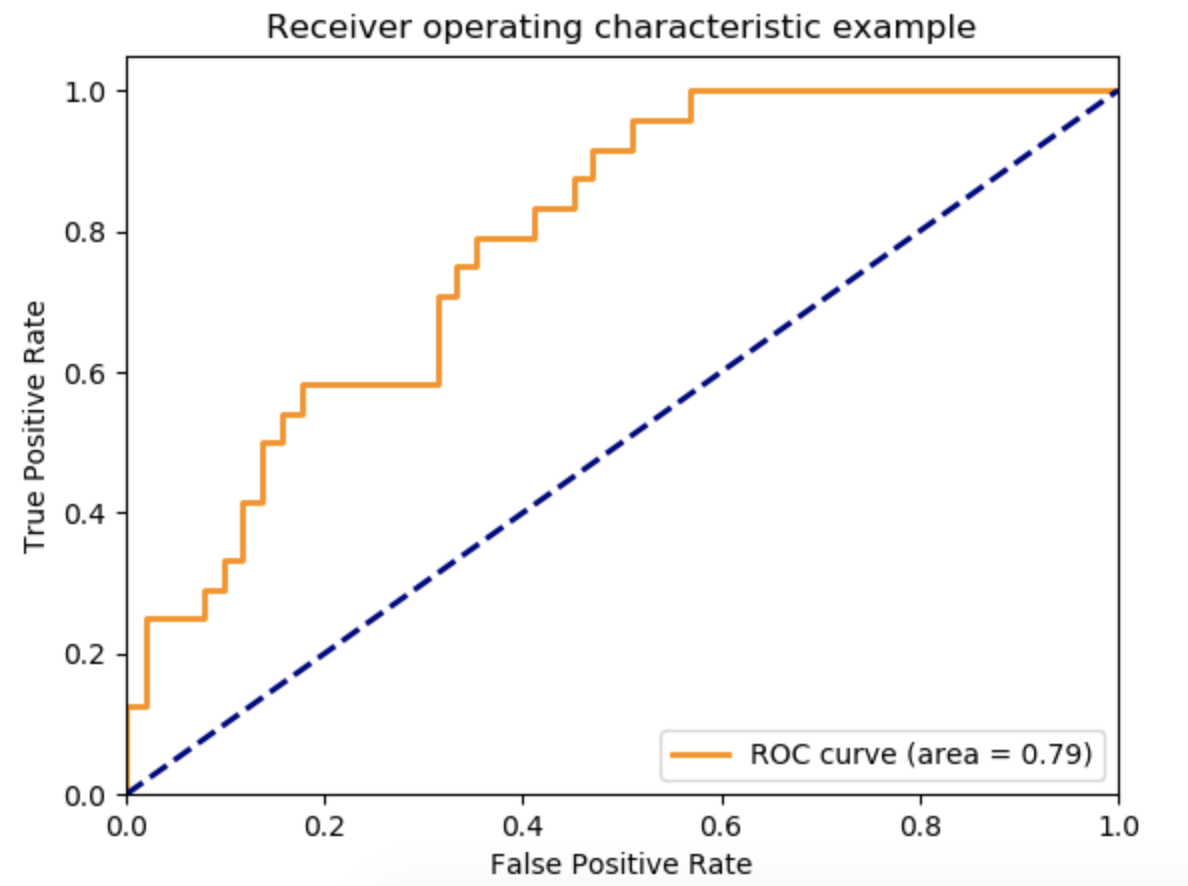pr 曲线从左到右是下降的，roc 曲线从左到右是上升的，个人认为二者皆可，不过大家普遍都采用 roc 曲线（上面两张图片都来自 scikit-learn 官方网站）

展开全文机器学习
• 深度学习 实验三 logistic回归预测二分类 学会使用学习到的逻辑回归的知识，手动使用梯度下降方法，通过给定的相关数据来完成年薪是否高于50k的二分类预测任务。

# 深度学习 实验三 logistic回归预测二分类

## 一、问题描述

学会使用学习到的逻辑回归的知识，手动使用梯度下降方法，通过给定的相关数据来完成年薪是否高于50k的二分类预测任务。

## 二、设计简要描述

相关文件：链接：https://pan.baidu.com/s/1FhWygBCq74EPMmbGCYwuYA 提取码：2lns

1. 数据预处理

1.1 读取数据

1.2 输入数据格式化处理

1.2.1 去除字符串数值前面的空格

方便后续字符串类型数据处理需要去掉字符串之前的空格。

1.2.2 对字符数据进行编码

对数据进行OneHot编码处理。

2. 数据标准化

将用于训练的数据进行标准化处理，这里是主要是将其进行正态分布化处理。

3. 逻辑回归模型实现

定义用于实现逻辑回归模型的各个功能的函数。

①　_sigmoid：计算输入的sigmoid；

②　get_prob：在给定权重和偏差的情况下，找出模型预测输出1的概率；

③　infer: 如果概率>为0.5，则输出1，否则输出0；

④　_crossentropy: 计算模型输出和真实标签之间的交叉熵；

⑤　_computeLoss : 计算输入为X, Y, w的损失函数L(w) ；

⑥　_gradient_regularization : 通过数学推导损失函数；

⑦　train_dev_split: 按照 dev_size 的比例分割数据，用于使用交叉验证时的情况；

⑧　_shuffle：打乱一列原来的顺序；

⑨　accuracy：精确度计算。

4. 划分训练集和验证集

利用数据分割函数将给定的训练集划分为测试集和验证集。

5. 训练模型

6. 验证集测试

利用得到的模型参数对验证集进行预测验证，查看准确率和损失值。

7. 测试集测试

对给定的测试集进行模型预测。

8. 保存预测结果到文件

将对预测集预测得到的结果按照要求格式保存到csv文件中。

## 三、程序清单

# 逻辑回归实现二分类任务：确定一个人是否年收入超过5万美元。
import pandas as pd
import numpy as np
import csv

# 1. 数据预处理
# 1.1 读取数据

# 1.2 输入数据格式化处理
# 1.2.1 去除字符串数值前面的空格
str_cols = [1,3,5,6,7,8,9,13,14]
for col in str_cols:
train_data.iloc[:,col] = train_data.iloc[:,col].map(lambda x: x.strip())
if col != 14:
test_data.iloc[:,col] = test_data.iloc[:,col].map(lambda x: x.strip())

# 1.2.2 对字符数据进行编码
from sklearn.preprocessing import MinMaxScaler
min_max_scaler = MinMaxScaler()
data = pd.concat([train_data,test_data],axis=0)
data[['age','fnlwgt' ,'education_num' ,'capital_gain' ,'capital_loss' ,'hours_per_week']]=min_max_scaler.fit_transform(data[['age','fnlwgt' ,'education_num' ,'capital_gain' ,'capital_loss' ,'hours_per_week']])

data.loc[data['workclass']=='?','workclass']='Private'
data.loc[data['occupation']=='?','occupation']='other'

data.loc[data['income']=='<=50K','income']='0'
data.loc[data['income']=='>50K','income']='1'

cols=['workclass', 'education','marital_status', 'occupation', 'relationship', 'race', 'sex', 'native_country']
for col in cols:
tmp=pd.get_dummies(data[col],prefix=col)
data=pd.concat([data,tmp],axis=1)
data=data.drop(col,axis=1)

train_data = data[0:train_data.shape]
test_data = data[train_data.shape:]

train_encoded_data = np.array(train_data.drop(columns='income'))
test_encoded_data = np.array(test_data.drop(columns='income'))

# 2.2.数据标准化
train_mean = np.mean(X_train,axis=0).reshape(1, -1)
train_std = np.std(X_train,axis=0).reshape(1,-1)

# 将指定列规格化为正态分布,以使模型更容易地了解数据分布。
X_train = np.divide(np.subtract(X_train,train_mean), train_std)

# 3.逻辑回归模型实现
# 计算输入的sigmoid
def _sigmoid(z):
result = np.clip(1 / (1.0 + np.exp(-z)), 1e-6, 1-1e-6)
return result

# get_prob: 在给定权重和偏差的情况下，找出模型预测输出1的概率
def get_prob(X, w, b):
y = _sigmoid(X.dot(w)+b)
return y

# infer: 如果概率>为0.5，则输出1，否则输出0。
def infer(X, w, b):
y = np.round(get_prob(X, w, b))
return y

# _crossentropy: 计算模型输出和真实标签之间的交叉熵。
def _crossentropy(y_pred, y_label):
#     y_label = int(y_label)
#     loss = np.sum(np.nan_to_num(-y_label*np.log(y_pred)-(1-y_label)*np.log(1-y_pred)))
Y = np.float_(y_label)
P = np.float_(y_pred)
loss = -np.sum(Y * np.log(P) + (1 - Y) * np.log(1 - P))
return loss

# _computeLoss : 计算输入为X, Y, w的损失函数L(w)
def _computeLoss(y_pred, Y_label, lamda, w):
loss = 0
for i in range(len(y_pred)):
loss += _crossentropy(y_pred[i], Y_label[i]) + lamda * np.sum(np.square(w))
#         loss += (y_pred[i] - int(Y_label[i]))**2
return loss

# _gradient_regularization : 通过数学推导损失函数
def _gradient_regularization(X, Y, w, b, lamda):
#print(X.shape)
pred_error = np.ones(X.shape)
for i in range(X.shape):
pred_error[i] = (Y[i] - w.dot(X[i]) - b) * (-1)
w_grad = -np.mean(np.multiply(pred_error.T, X.T), 1) + lamda*w

# train_dev_split: 按照 dev_size 的比例分割数据，用于使用交叉验证时的情况
def train_dev_split(X, y, dev_size=0.2):
train_len = int(round(len(X)*(1-dev_size)))
return X[0:train_len], y[0:train_len], X[train_len:None], y[train_len:None]

# _shuffle:打乱一列原来的顺序
def _shuffle(X, Y):
randomsize = np.arange(len(X))
np.random.shuffle(randomsize)
return (X[randomsize], Y[randomsize])

# accuracy：精确度计算
def accuracy(Y_pred, Y_label):
Y_label = list(map(int,Y_label))
acc = np.sum(Y_pred == Y_label)/len(Y_label)
return acc

# 4.划分训练集和验证集
X, y = train_encoded_data, np.array(train_data['income'])
X_train,y_train, X_val, y_val = train_dev_split(X, y)

# 5.训练过程
max_iter = 30
batch_size = 25
lr = 0.1

# 正则项系数
lamda = 0.001

w = np.ones(len(X_train))
b = 0

train_acc = []
loss_train = []

step = 1

for epoch in range(max_iter):
# 打乱每次训练的数据
X_train, y_train = _shuffle(X_train, y_train)

# 逻辑回归按批次训练
for idx in range(int(np.floor(len(y_train)/batch_size))):
X = X_train[idx*batch_size:(idx+1)*batch_size]
Y = y_train[idx*batch_size:(idx+1)*batch_size]
Y = list(map(int,Y))

# 计算梯度损失

# 梯度更新
w -= lr / np.sqrt(step) * w_grad
b -= lr / np.sqrt(step) * b_grad

step += 1

# print(w, b)
# 在每个epoch训练中记录下训练误差 以及验证集中的误差用于画图数据
y_train_pred = get_prob(X_train, w, b)
Y_train_pred = np.round(y_train_pred)
loss_train.append(_computeLoss(y_train_pred, y_train, lamda, w)/len(X_train))
train_acc.append(accuracy(Y_train_pred, y_train))

#     if epoch%5 == 0:
print('epoch {}, the loss is : {}, the acc is : {}'.format(epoch+1, loss_train[epoch], train_acc[epoch]))

# 6.验证集测试结果
y_val_pred = get_prob(X_val, w, b)
Y_val_pred = np.round(y_val_pred)
loss_val = _computeLoss(y_val_pred, y_val, lamda, w)/len(X_val)
val_acc = accuracy(Y_val_pred, y_val)
print('validation data, the loss is : {}, the acc is : {}'.format(loss_val, val_acc))

# 7.测试集测试
Y_test_pred = infer(test_encoded_data, w, b)
Y_test_pred = list(map(int,Y_test_pred))

# 8.保存预测结果到文件
predict_result_file = open('./predict_result.csv','w',newline='')
writer = csv.writer(predict_result_file)
writer.writerow(('id','label'))
for i in range(len(Y_test_pred)):
writer.writerow([i+1, Y_test_pred[i]])
predict_result_file.close()

展开全文logistic回归
• 预测模型== 1.1 神经网络预测 1.2 灰色预测 1.3 拟合、插值预测（线性回归） 1.4 时间序列预测 1.5 马尔科夫链预测 1.6 微分方程预测 1.7 Logistic 回归（逻辑回归） 1.8 线性回归 总结 应用场景： ==2. 分类模型==...matlab Python
• 表5-9中，已知前20家企业的三项评价指标值和评估结果，试建立神经网络，决策树模型对剩余5家企业进行评估。 (数据下载，无需积分) 解题过程： 建立双层前向神经网络模型： 编写matlab代码，构造一个输入结点...神经网络 机器学习 matlab
• ## python简单预测模型

千次阅读 2021-02-04 08:49:02
python简单预测模型步骤1：导入所需的库，读取测试和训练数据集。#导入pandas、numpy包，导入LabelEncoder、random、RandomForestClassifier、GradientBoostingClassifier函数import pandas as pdimport numpy as np...
• 需求预测（demand forecast） 我们在构建模型时，主要分三步： 查看input data ...第步针对input data，我们需要根据需求确定合适的metrics，比如针对需求预测准确度的accuracy指标我们有MAPE, APE, AE, RM...
• ## 类别预测模型

千次阅读 2020-02-13 20:02:10
类别预测模型概述 自变量是连续的；而因变量是分类变量。 如果两个自变量，对应就是维的； 因变量，作为分类变量，可以是两类，也可以是多类，这里，简单地，以两类为例进行分析。 上图中，横坐标对应自变量x1x_{...
• ## 2.二分类模型

万次阅读 2019-05-08 22:03:57
逻辑回归：一个用于二分类的算法 以猫的识别为例 图片（64X64像素）在计算机中的保存：三个64X64的矩阵,对应R,G,B三种像素的强度值。定义一个特征向量，线性存储所有像素值，总维度是64X64X3。 输入...吴恩达 二分类
• 原本使用了logitic逻辑回归模型 预测了结果 但没有概率 后来尝试借鉴大佬的手撸代码 结果不太对 后来又学习到大佬的tensorflow神经网络 可以正确预测出概率 并画出正确率图，且正确率很高 数据集 data_horse.csv...tensorflow 神经网络 机器学习 人工智能
• 目录 九、预测模型 1.灰色预测模型 2.BP神经网络 十、降维模型 1.奇异值分解（Singular Value ...预测模型主要涉及灰色预测模型、神经网络预测模型。 1.灰色预测模型 ...机器学习 神经网络 matlab
• 使用Keras训练一个简单的LSTM二分类网络模型，用于找到数列中是否包含3个连续递增或者递减的子数列。比如 [ 0.1, 0.2, 0.3, 0.5, 0.3, 0.2 ] 数列对应的标签为[ 0, 0, 1, 1 , 0, 1 ]。 特诊 设 [x1, x2, x3]中x3的...神经网络 keras tensorflow
• 选两种花进行二分类。 import torch import numpy as np import pandas as pd import torch.nn as nn import matplotlib.pyplot as plt import torch.nn.functional as F from torch.utils.data import Dataset ...
• 问题建模 机器学习解决问题的通用流程...在这个过程中要明确业务指标和模型预测目标，根据预测目标选择适当指标用于模型评估。接着从原始数据中选择最相关的样本子集用于模型训练，并对样本子集划分训练集和测试集...
• 预测模型的分解过程 我总是集中于投入有质量的时间在建模的初始阶段，比如，假设生成、头脑风暴、讨论或理解可能的结果范围。所有这些活动都有助于我解决问题，并最终让我设计出更强大的商业解决方案。为什么你要在...
• ## 预测模型评估

千次阅读 2019-07-22 16:11:14
偏差描述的是模型预测准不准，低偏差就是表示模型预测能力是不错的，就像图中的点都在靶心附近。 方差描述的是模型稳不稳定，就像图中高方差的那些点，它们很分散，说明射击的成绩不稳定，波动很大。 、用学习...
• 临床预测模型是指使用参数/半参数/非参数数学模型来评估受试者当前患有某种疾病的概率或将来发生某种结局的可能性。通过该模型，利用已知特征来计算未知结局发生的概率。临床预测模型一般采用各种回归分析方法建模...
• 通常通过绝对/相对误差、平均绝对误差、均方误差、均方根误差等指标来衡量模型预测效果。 (1)绝对误差与相对误差 Y表示真实值，Y^表示预测值 E为绝对误差：E=Y-Y^ e为相对误差：e=(Y-Y^)/Y (2)平均绝对误差(Mean ...
• Python深度学习笔记（三）二分类模型 继续来写我的学习笔记 -----二分类问题 我从数据的处理，模型的构架，训练过程，验证模型及损失和精度的比较这几个方面来介绍。 一，数据的处理 一个典型的二分类问题的例子：...
• ## 常见的预测模型及算法

万次阅读 多人点赞 2020-05-01 13:22:18
如果得到一份数据集，任务是要预测出一系列的值，而在预测任务中，我们大多数都采用的是拟合的方法，这篇文字主要介绍三种预测方法时间序列分析，灰色预测模型，神经网络。 时间序列分析 时间序列也叫动态序列，数据...
• 01前言02分类问题的解决本实战部分所使用的数据集是关于手体字母的识别，当一个用户在设备中写入某个字母后，该设备就需要准确地识别并返回写入字母的实际值。...接下来利用SVM模型对该数据集的因变量...
• ## 几种常见的预测模型

万次阅读 多人点赞 2018-03-26 16:14:42
几种常见的预测模型1.趋势外推预测方法趋势外推预测方法是根据事物的历史和现实数据，寻求事物随时间推移而发展变化的规律，从而推测其未来状况的一种常用的预测方法。 趋势外推法的假设条件是： (1)假设事物发展...数据预测
• 有人说21世纪，我们的生活越来越便捷，电子通讯越来越发达，美食越来越多。...人工智能机器学习可以帮助医药科研人员挖掘更多糖尿病致病因子，建立模型预测患者血糖。 欢迎各位同学学习python机器...python 人工智能 机器学习
• 二分类模型数量众多，但实际应用中往往待预测类别数量不只有2个，于是有了一些将二分类模型应用到多分类的方法。 常见二分类模型 One-vs-Rest One-vs-One Directed Acyclic Graph Method 常见二分类模型 ...
• 之前设置了0.1， 0.01，0.0001，0.00001进行训练，但全数据集全部预测到了同一类。 检查 从权重开始检查，发现学习率为0.1的时候，第一个epoch的模型权重就已经很小很小了，目前猜测是学习率太大，现在从0.0000001...pytorch
• knitr::opts_chunk\$set(echo = TRUE)  主要介绍二分类评价的一些指标。 混淆矩阵   预测为正类 预测为负类 实际为正类 TP FN 实际为负类 FP TN 符号标记： TP—将正类预测为正类数 FN—将正类预测为负...
• 1）实例化模型 Assume that the content ofYourClass.pyis: class YourClass: # ...... If you use: from YourClassParentDir import YourClass # means YourClass from model import PythonNet ...pytorch 神经网络 图像分类预测
• SPSS项logistic回归分析案例实践，做个预测模型自己动手实践是学习统计软件工具的捷径之一。收集到某公司各个商户id，以及他们的注册时长、营业收入、成本数据，以及合作续约的情况，现在我们想尝试基于这样的数据...
• 二分类预测用的几个预测结果精确度计算方法 # Print accuracy predictions = predict(parameters, X) # (np.dot(Y, predictions.T) + np.dot(1 - Y, 1 - predictions.T)) / Y.size print (......